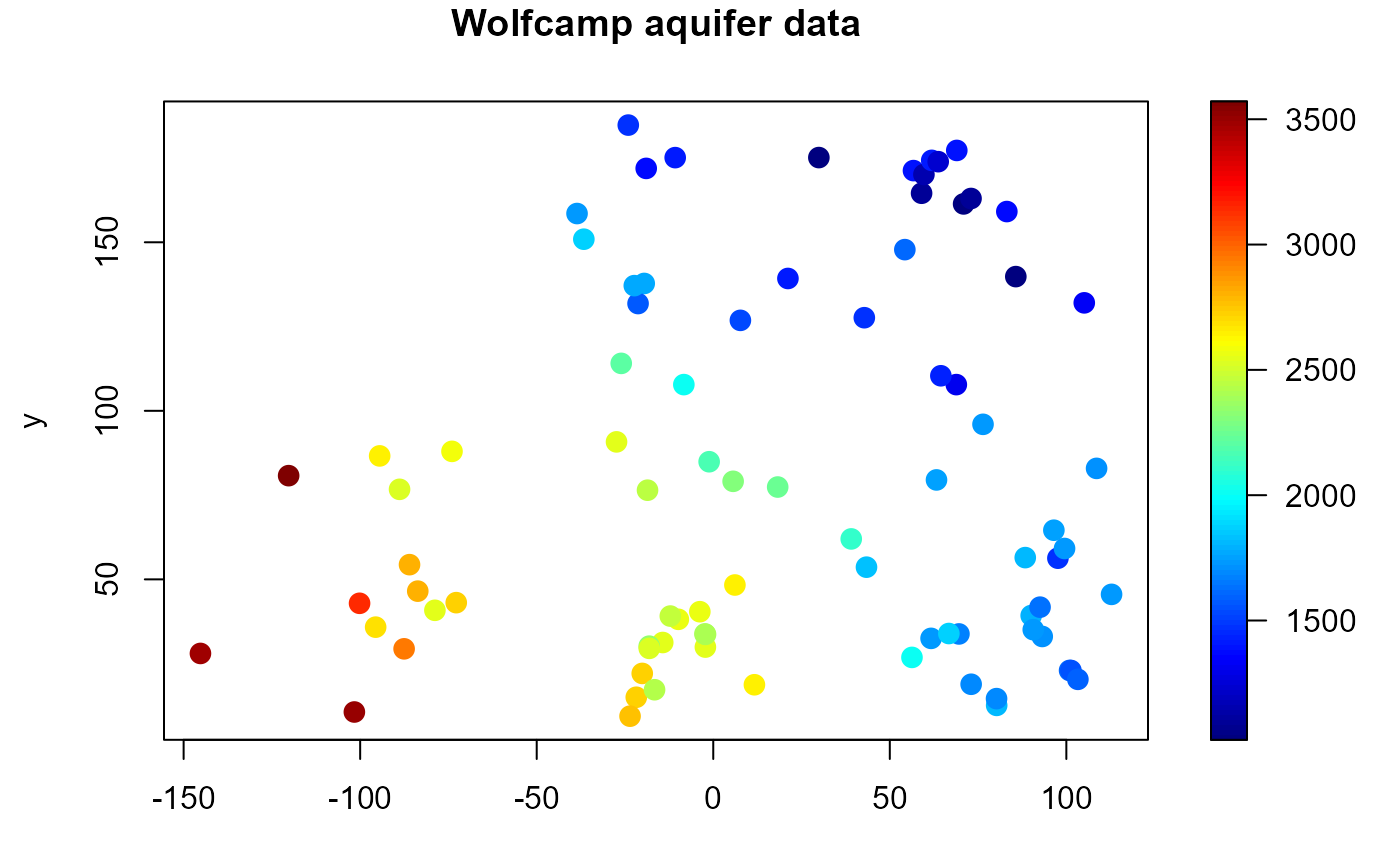spoints (generic function) draws a scatter plot with points filled with different colors and (optionally) adds a legend strip with the color scale (calls splot and plot.default).

spoints(x, ...)

# S3 method for default
spoints(
x,
y = NULL,
s,
slim = range(s, finite = TRUE),
col = jet.colors(128),
breaks = NULL,
legend = TRUE,
horizontal = FALSE,
legend.shrink = 1,
legend.width = 1.2,
legend.mar = ifelse(horizontal, 3.1, 5.1),
legend.lab = NULL,
bigplot = NULL,
smallplot = NULL,
lab.breaks = NULL,
axis.args = NULL,
legend.args = NULL,
pch = 16,
cex = 1.5,
xlab = NULL,
ylab = NULL,
asp = NA,
...
)

# S3 method for data.grid
spoints(x, s = x[], xlab = NULL, ylab = NULL, ...)

# S3 method for SpatialPointsDataFrame
spoints(x, data.ind = 1, main, xlab, ylab, legend.lab, ...)

## Arguments

x object used to select a method. In the default method, it provides the x coordinates for the plot (and optionally the y coordinates; any reasonable way of defining the coordinates is acceptable, see the function xy.coords for details). additional graphical parameters (to be passed to the main plot function or sxxxx.default; e.g. xlim, ylim, ...). NOTE: graphical arguments passed here will only have impact on the main plot. To change the graphical defaults for the legend use the par function beforehand (e.g. par(cex.lab = 2) to increase colorbar labels). y coordinates. Alternatively, a single argument x can be provided. numerical vector containing the values used for coloring the points. limits used to set up the color scale. color table used to set up the color scale (see image for details). (optional) numeric vector with the breakpoints for the color scale: must have one more breakpoint than col and be in increasing order. logical; if TRUE (default), the plotting region is splitted into two parts, drawing the main plot in one and the legend with the color scale in the other. If FALSE only the (coloured) main plot is drawn and the arguments related to the legend are ignored (splot is not called). logical; if FALSE (default) legend will be a vertical strip on the right side. If TRUE the legend strip will be along the bottom. amount to shrink the size of legend relative to the full height or width of the plot. width in characters of the legend strip. Default is 1.2, a little bigger that the width of a character. width in characters of legend margin that has the axis. Default is 5.1 for a vertical legend and 3.1 for a horizontal legend. label for the axis of the color legend. Default is no label as this is usual evident from the plot title. plot coordinates for main plot. If not passed, and legend is TRUE, these will bedetermined within the function. plot coordinates for legend strip. If not passed, and legend is TRUE, these will be determined within the function. if breaks are supplied these are text string labels to put at each break value. This is intended to label axis on a transformed scale such as logs. additional arguments for the axis function used to create the legend axis (see image.plot for details). arguments for a complete specification of the legend label. This is in the form of list and is just passed to the mtext function. Usually this will not be needed (see image.plot for details). logical; if TRUE the scatter plot is just added to the existing plot. logical; if FALSE (default) the plotting region (par("plt")) will not be reset to make it possible to add more features to the plot (e.g. using functions such as points or lines). If TRUE will reset plot parameters to the values before entering the function. vector of plotting characters or symbols: see points. numerical vector giving the amount by which plotting characters and symbols should be scaled relative to the default. This works as a multiple of par("cex"). label for the x axis, defaults to a description of x. label for the y axis, defaults to a description of y. the y/x aspect ratio, see plot.window. integer (or character) with the index (or name) of the data component. an overall title for the plot.

## Value

Invisibly returns a list with the following 3 components:

bigplot

plot coordinates of the main plot. These values may be useful for drawing a plot without the legend that is the same size as the plots with legends.

smallplot

plot coordinates of the secondary plot (legend strip).

old.par

previous graphical parameters (par(old.par) will reset plot parameters to the values before entering the function).

## Details

spoints.SpatialPointsDataFrame sets default values for some of the arguments from attributes of the object x (if present; see e.g. precipitation).

## Side Effects

After exiting, the plotting region may be changed (par("plt")) to make it possible to add more features to the plot (set graphics.reset = FALSE to avoid this).

splot, simage, spersp, image, image.plot, data.grid, plot.default.

## Author

Based on image.plot function from package fields: fields, Tools for spatial data. Copyright 2004-2013, Institute for Mathematics Applied Geosciences. University Corporation for Atmospheric Research.

Modified by Ruben Fernandez-Casal <rubenfcasal@gmail.com>.

## Examples


#
with( aquifer, spoints(lon, lat, head, main = "Wolfcamp aquifer data"))